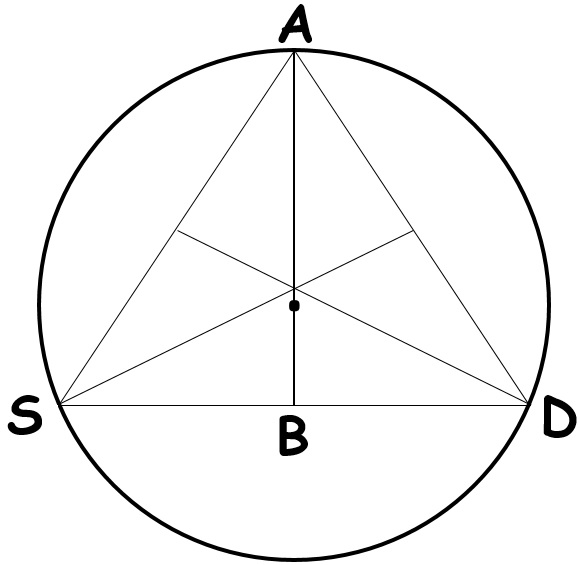# vs.eyeandcontacts.com

## Chapter 10 Circles Exercise 10.4

Question 1: Two circles of radii 5 cm and 3 cm intersect at two points and the distance between their centres is 4 cm. Find the length of the common chord.Given:
Two intersecting circles (with radius 5cm and 3cm).
Distance between their centres, AB, is 4cm.
EF is the common chord.
AD = BC = 1 cm
BD = 3cm
AE = 5cm

Required to find:
The length of the common chord, EF.

Proof:
We know that the line joining the centres of two intersecting circles bisect the common chord perpendicularly.

Hence (triangle) ABE forms a right angled triangle.

Using pythagoras theorem,
AE² = AB² + BE²
= (5)² = (4)² + BE²
= √(25 - 16) = BE
= √9 = BE or BE = 3cm

As AC bisects common chord EF perpendicularly
(therefore) EF = 2BE or EF = 6cm.

Question 2: If two equal chords of a circle intersect within the circle, prove that the segments of one chord are equal to corresponding segments of the other chord.Given:
2 equal chords of a circle (AB, CD) intersecting at P.

Required to prove:
CP = BP and AP = DP

Proof:
We have to draw OQ ⊥ CD and OR ⊥ AB.

We know that the perpendicular drawn from the centre of a circle to a chord bisects the chord.
Therefore, AR = BR = 1/2 AB and CQ = DQ = 1/2 DC.

As AB = CD
→ 1/2 AB = 1/2 CD
→ AR = DQ    …(1)
and CQ = BR    …(2)

Join OP
In triangle OPQ and triangle OPR,
OP = OP (common side)
angle PQO = angle PRO (each 90)
OQ = OR (equal chords [AB and CD] equidistant from the centre)

Therefore triangle OPQ ≅ OPR (RHS rule)
By CPCT, PQ = PR

PQ = PR
CQ + PQ = CQ + PR (adding CQ to both sides)
PC = PQ + RB (from …(2))
PC = PB …(3)

CD - PC = PD
AB - BP = PD
PA = PD …(4)

From (3) and (4) PC = PB and PA = PD

Question 3: If two equal chords of a circle intersect within the circle, prove that the line joining the point of intersection to the centre makes equal angles with the chords.Given:
2 equal chords of a circle (AB, CD) intersecting at P.

Required to prove:
CP = BP and AP = DP

Proof:
We have to draw OQ ⊥ CD and OR ⊥ AB

We know that the equal chords of a circle are at equal distance from the centre of the circle.
Therefore, AR = BR = 1/2 AB and CQ = DQ = 1/2 DC.

As AB = CD
→ 1/2 AB = 1/2 CD
→ AR = DQ    …(1)
and CQ = BR    …(2)

Join OP
In triangle OPQ and triangle OPR,
OP = OP (common side)
angle PQO = angle PRO (each 90)
OQ = OR (equal chords [AB and CD] equidistant from the centre)

Therefore triangle OPQ ≅ OPR (RHS rule)
By CPCT, PQ = PR

Question 4: If a line intersects two concentric circles (circles with the same centre) with centre O at A, B, C and D, prove that AB = CD (see Fig. 10.25).We know that the perpendicular drawn from the centre of a circle to a chord bisects the chord.
Therefore,
AB = CD
BM = MC
AM = MD
AM - BM = MD - MC → AB = CD

Hence proved.

Question 5: Three girls Reshma, Salma and Mandip are playing a game by standing on a circle of radius 5m drawn in a park. Reshma throws a ball to Salma, Salma to Mandip, Mandip to Reshma. If the distance between Reshma and Salma and between Salma and Mandip is 6m each, what is the distance between Reshma and Mandip?Draw perpendiculars OA and OB on RS and SM respectively.

Therefore, AR = AS = 6/2 m = 3m.

OR = OS = OM = 5m (radii of circle)

In triangle OAR,
OA² + AR² = OR²
OA² + (3m)² = (5m)²
OA = √(25 - 9)
OA = 4m

ORSM is a kite as adjacent pair of sides are equal (OR = OM and RS = SM). We know that the diagonals of a kite are perpendicular and the diagonal common to both the isosceles triangles is bisected by another diagonal.

→ ∠RCS will be 90° and RC = CM.

Area of triangle ORS = 1/2 x OA x RS
1/2 x RC x OS = 1/2 x 4 x 6
RC x 5 = 24
RC = 24/5 or RC = 4.8m

RM = 2RC
RM = 9.6m

Question 6: A circular park of radius 20m is situated in a colony. Three boys Ankur, Syed and David are sitting at equal distance on its boundary each having a toy telephone in his hands to talk each other. Find the length of the string of each phone.It is given that AS = SD = DA.

Therefore, triangle ASD is an equilateral triangle.

We know that medians of equilateral triangle pass through the circumference of the equilateral triangle ASD. We also know that the medians intersect in the ration of 2:1. As AB is the median of equilateral triangle ASD we can write,

= OA/OB = 2/1
= 20/OB = 2/1
= OB = 10m

AB = OA + OB
AB = 20 + 10 = 30m

In triangle ABD,